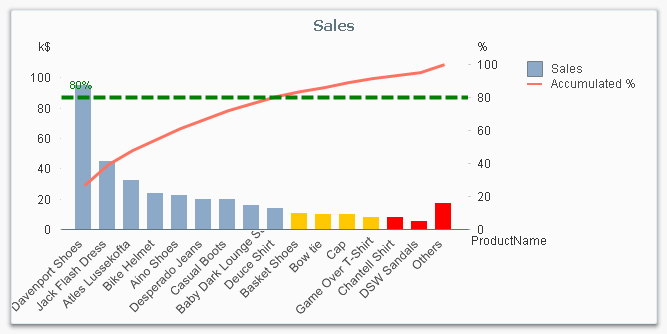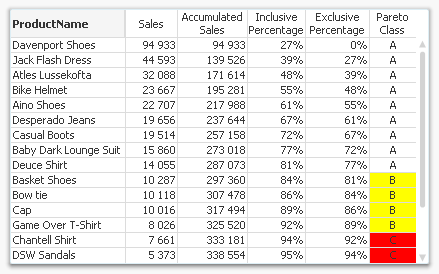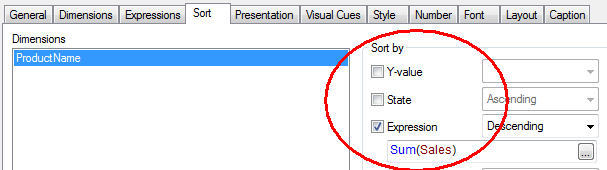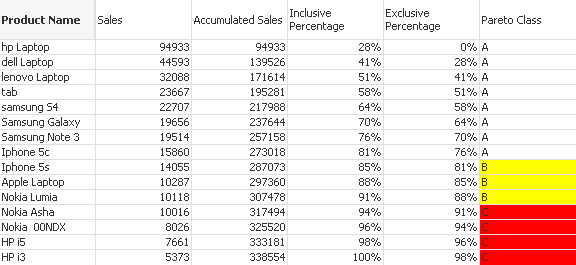# Qlik Design Blog

All about product and Qlik solutions: scripting, data modeling, visual design, extensions, best practices, etc.Employee

## Recipe for a Pareto Analysis

“Which products contribute to the first 80% of our turnover?”

This type of question is common in all types of business intelligence. I say “type of question” since it appears in many different forms: Sometimes it concerns products, but it can just as well concern customers, suppliers or sales people. It can really be any dimension. Further, here the question was about turnover, but it can just as well be number of support cases, or number of defect deliveries, etc. It can in principle be any additive measure.

It is called Pareto analysis. Sometimes also known as 80/20 analysis or ABC analysis.

The logic is that you first sort the products according to size, then accumulate the numbers, and finally calculate the accumulated measure as a percentage of the total. The products contributing to the first 80% are your best products; your “A” products. The next 10% are your “B” products, and the last 10% are your “C” products.And here’s how you do it in QlikView:

1. Create a pivot table and choose your dimension and your basic measure. In my example, I use Product and Sum(Sales).

2. Sort the chart descending by using the measure Sum(Sales) as sort expression. It is not enough just to check “Sort by Y-value”.

3. Add a second expression to calculate the accumulated sales value:
RangeSum(Above(Sum(Sales), 0, RowNo()))
Call this expression Accumulated Sales. The Above() function will return an array of values – all above values in the chart – and the RangeSum() function will sum these numbers.

4. Create a third expression from the previous one; one that calculates the accumulated sales in percent:
RangeSum(Above(Sum(Sales), 0, RowNo())) / Sum(total Sales)
Format it as a percentage and call it Inclusive Percentage.

5. Create a fourth expression from the previous one; one that calculates the accumulated sales in percent, but this time excluding the current row:
RangeSum(Above(Sum(Sales), 1, RowNo())) / Sum(total Sales)
Format it as a percentage and call it Exclusive Percentage.

6. Create a fifth expression for the ABC classification:
If([Exclusive Percentage] <= 0.8, 'A', If([Exclusive Percentage] <= 0.9, 'B', 'C'))
Call this expression Pareto Class. The reason why the Exclusive Percentage is used, is that the classification should be determined by the lower bound of a product’s segment, not the upper.

7. Create a conditional background color, e.g.
If([Pareto Class] = 'C', LightRed(), If([Pareto Class] = 'B', Yellow()))

You should now have a table similar to the following. In it you can clearly see the classification of different products.In this table, there are five different expressions that you can use for Pareto analysis. The graph in the beginning of this post uses Sales and Inclusive Percentage for the bars and the line, respectively; and Pareto Class for the coloring of the bars.

Further, you may want to combine the Pareto Class and the Exclusive Percentage into one expression:

Pareto Class =
If(RangeSum(Above(Sum(Sales),1,RowNo())) / Sum(total Sales) <= 0.8, 'A',
If(RangeSum(Above(Sum(Sales),1,RowNo())) / Sum(total Sales) <= 0.9, 'B', 'C'))

Good luck in creating your Pareto chart.

HIC

Further reading related to this topic:

Recipe for an ABC Analysis

Recipe for a Pareto Analysis – Revisited

127 CommentsSpecialist II

Very useful and easy to understand article.

Thanks Henric.....

15,632 ViewsMVP

Useful Post

15,631 ViewsNot applicable

Very great as always - And i like that you have given an example in steps. Even understandable for the biggest rookieKeep em coming!

Thumps up!

15,632 ViewsPartner

Really useful. I was looking for a method to calculate the ABC sections and although I haven't found a way to calculate that in the fly (i.e. without and accumulated chart)... at least this method looks nice.

15,632 ViewsEmployee

Also I have tried to find a way to calculate the Pareto class on the fly (so you can use this as a calculated dimension), but have not succeeded. So I don't think it is possible, given the existing set of functions. But having it as expression is possible, as I have shown in the post above.

HIC

Edit: The above answer was correct when it was made, but things have changed. Now it is possible to calculate the Pareto class as dimension. See Recipe for a Pareto Analysis – Revisited

15,632 ViewsNot applicable

Hi Henric,

Pareto Analysis is Excellent task .I am not getting Accumulated Sales value . i am using the Same Expression Which u have used .Can u help me

Thanks in Advance

15,632 ViewsNot applicableIf  I use the Expression then i m getting the above values .May i know the reason .

9,868 ViewsEmployee

Without the data, I can only guess. And my guess is that you have not used a sort expression to sort the rows of the chart. (The second bullet in the description above.) It does not work if you just sort by y.

HIC9,868 ViewsChampion

Very useful and easy to understand article.

Thanks Henric....9,868 ViewsNot applicable

Hi Henric ,

Your guess is Absolutely Correct .I have used same data which u have used in this article .i am getting different Inclusive and Exclusive Percentages . Can u please help me and can u tell me step by step Process for Creating Chart which u have created in  article.Thanks for Your Response

Regards,

Divya

9,868 Views